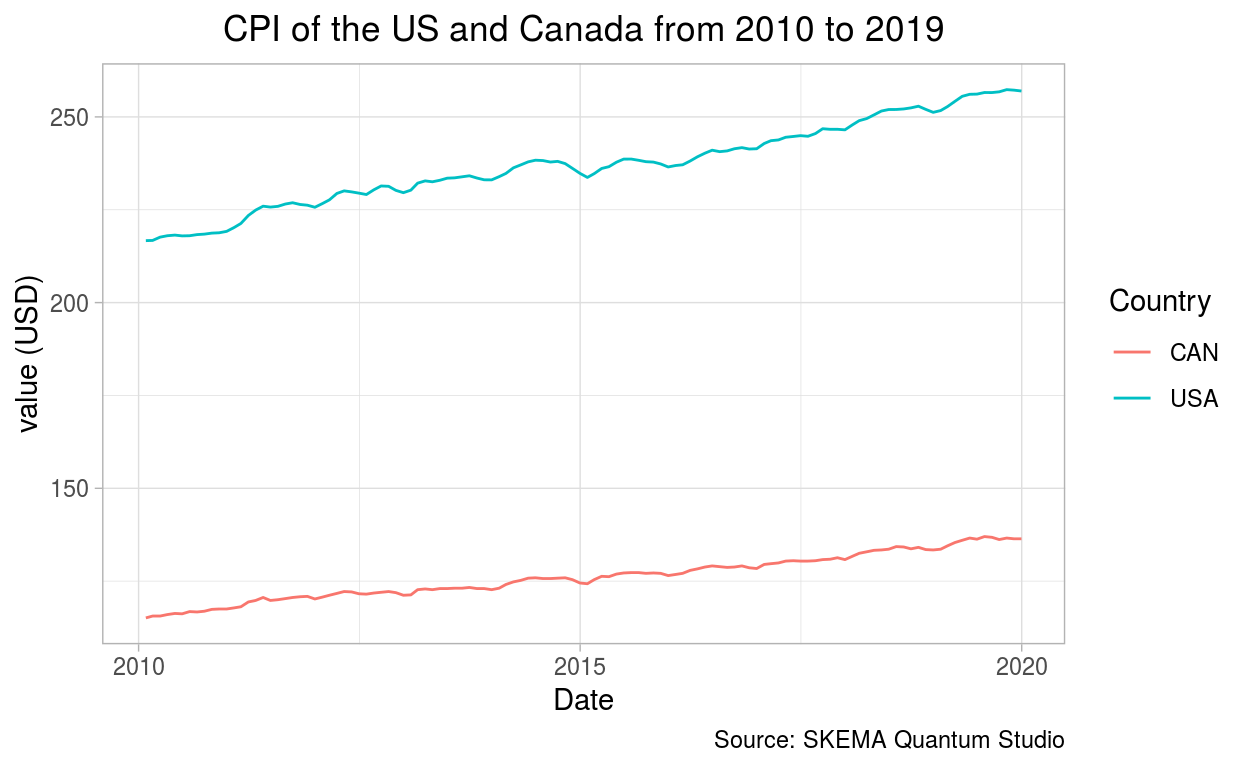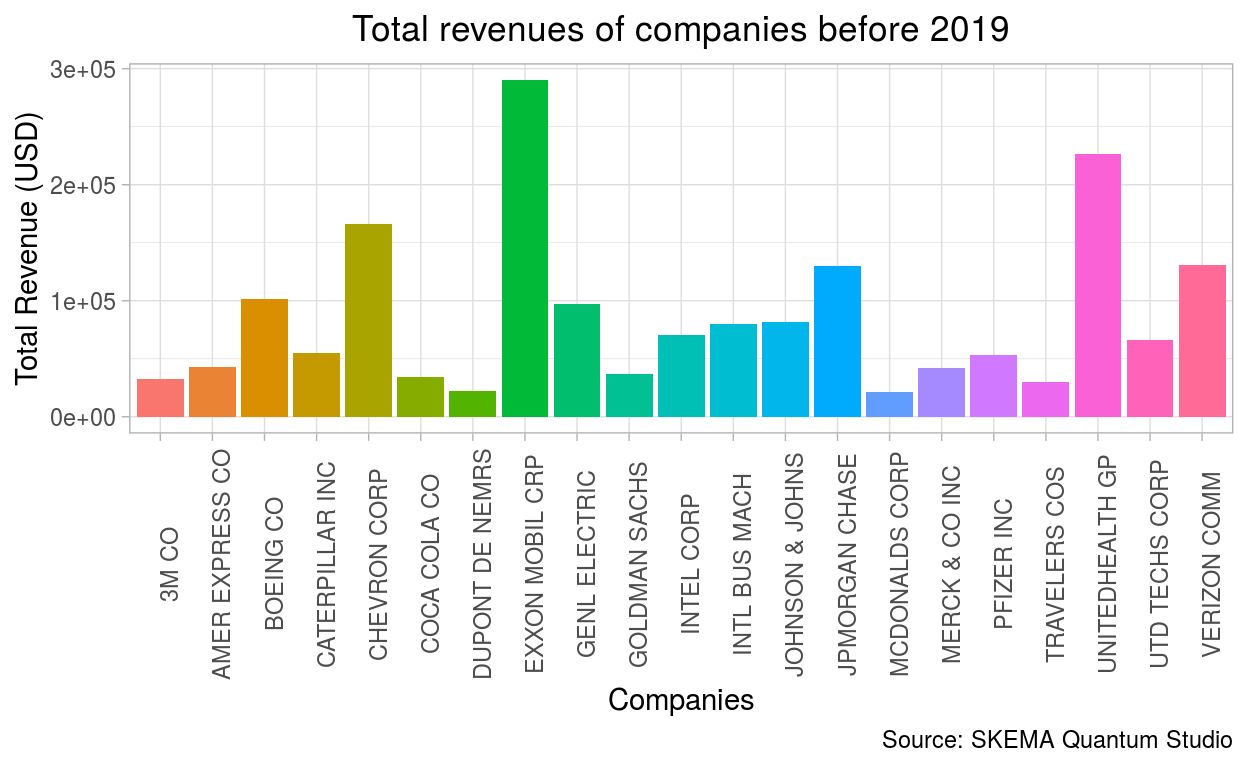# Quandl

Extract value from the world’s data

Anne Sophie Gill https://www.skemagloballab.io/gillAnneSophie.html , Marine Leroi https://www.skemagloballab.io/leroiMarine.html (SKEMA Global Lab in AI)https://skemagloballab.io , Thierry Warin https://www.nuance-r.com/principalInvestigator.html (SKEMA Business School (Raleigh, NC))https://www.skemagloballab.io
02-19-2020

Using the SKEMA Quantum Studio(Warin 2019) framework, we will teach you how to use the Quandl package

Just like the SKEMA Global Lab in AI, the Quandl team believes data is one of the most important resources of the 21st century. Their mission is to extract value from the world’s data. To do so, Quandl collects financial and economic datasets from hundreds of publishers on one convenient platform.

## How to use the Quandl package

### Step One : Register your API key


library(Quandl)

# Enter your API key in the function "Quandl.api_key"
# Quandl.api_key("abcdefghijklmnopqrstuvwxyz123456") 

Before using the Quandl package, you have to (freely) register to the institution.

After confirming your email adress to validate the key, you will have to enter your API key in the function Quandl.api_key(). This will take the API Key given by Quandl and will allow the access to the database.

### Step Two : Extract the data

Quandl() function


# The Consumer Price Index of the US from 2010 to 2019

mydata_usa = Quandl("RATEINF/CPI_USA", start_date="2010-01-01", end_date="2019-12-31")

# The Consumer Price Index of Canada from 2010 to 2019

mydata_can = Quandl("RATEINF/CPI_CAN", start_date="2010-01-01", end_date="2019-12-31")

Quandl.datatable() function


# The total revenues of companies before 2019

mydatafun = Quandl.datatable("ZACKS/FC", per_type="A", per_end_date="2018-12-31", qopts.columns=c("m_ticker", "comp_name","currency_code", "tot_revnu", "per_type", "per_end_date"))

### Step Three : Visualize your data

The Consumer Price Index (line chart)


# Data Wrangling : add a new column

library(dplyr) # load the "dplyr" library

mydata_usa$Country <- "USA" # add column "Country" and each variable in the column will be named USA mydata_can$Country <- "CAN" # add column "Country" and each variable in the column will be named CAN

df_new <- bind_rows(mydata_can, mydata_usa) # merge the 2 data frames together using the "bind_rows" function

library(ggplot2)

ggplot(data = df_new, aes(x = Date, y = Value, color = Country)) +
geom_line() +
theme_light() +
theme(plot.title = element_text(hjust = 0.5)) +
labs(title = "CPI of the US and Canada from 2010 to 2019",
x = "Date",
y = "value (USD)",
colour = "Country",
caption = "Source: SKEMA Quantum Studio")Total revenues of companies before 2019 (bar chart)


library(ggplot2)

ggplot(data = mydatafun, aes(x = comp_name, y = tot_revnu, fill = comp_name)) +
geom_col() +
theme_light() +
theme(plot.title = element_text(hjust = 0.5)) +
labs(title = "Total revenues of companies before 2019",
x = "Companies",
y = "Total Revenue (USD)",
caption = "Source: SKEMA Quantum Studio") +
theme(axis.text.x = element_text(margin = margin(t = .1, unit = "cm"), angle = 90)) +
theme(legend.position = "none")## A more complex example


library(Quandl)
library(dplyr)
library(spdep)
library(maptools)
library(leaflet)
library(maps)
library(rgdal)

# Enter your API key in the function "Quandl.api_key"
# Quandl.api_key("abcdefghijklmnopqrstuvwxyz123456")

# Extract your data from the Quandl package
JobUSA = Quandl.datatable("JL/JD2")

# Data Wrangling

names(JobUSA)[names(JobUSA) == "location_name"] <- "location" #rename column
names(JobUSA)[names(JobUSA) == "job_title"] <- "job" #rename column
names(JobUSA)[names(JobUSA) == "organization_country"] <- "country" #rename column
names(JobUSA)[names(JobUSA) == "region_label"] <- "NAME" #rename column
names(JobUSA)[names(JobUSA) == "organization_city"] <- "city" #rename column

JobUSA$country <- gsub("US", "USA", JobUSA$country) #change the term US for USA in the "country" column

JobUSAsub<- dplyr::select(JobUSA, NAME, posting_count) # select only relevant data in your data frame

JobUSAagg <- aggregate(posting_count ~ NAME, data = JobUSAsub, sum) # use the aggregate function to sum up entries in the "NAME" column that are the same

USmap <- readOGR('cb_2018_us_state_500k.shp')

OGR data source with driver: ESRI Shapefile
Source: "/home/gilla/mondo/skemalab/blog/_posts/2020-02-19-quandl/cb_2018_us_state_500k.shp", layer: "cb_2018_us_state_500k"
with 56 features
It has 9 fields
Integer64 fields read as strings:  ALAND AWATER 

Shapefile link United States Census Bureau


# Use "Left join" function to combine your two data frames

USmap@data <- left_join(USmap@data, JobUSAagg, by = "NAME")

# Create labels

USmap@data$NAME <- as.character(USmap@data$NAME)

USmap@data$posting_count <- as.numeric(USmap@data$posting_count)

labels <- sprintf("<strong>%s</strong><br/>%g", USmap@data$NAME, USmap@data$posting_count) %>% lapply(htmltools::HTML)

# Determinate the intervalls that will be shown in the map legend
bins <- c(0, 5, 10, 15, 20, 30, 40, 50, 60, 70)

# Choose a color scheme for your map

colors <- colorBin("Blues", domain = USmap@data$posting_count, bins = bins) # Plot the data using leaflet leaflet(USmap) %>% setView(lng = -95.712891, lat = 37.090240, zoom = 4) %>% addProviderTiles(providers$CartoDB.Positron) %>%
addLegend(pal = colors, values = USmap@data$posting_count, opacity = 0.7, title = NULL, position = "bottomleft") %>% addPolygons(fillColor = ~colors(USmap@data$posting_count),
weight = 2,
opacity = 1,
color = "white",
dashArray = 1,
fillOpacity = 0.8,
highlight = highlightOptions(weight = 2,
color = "black",
dashArray = 1,
fillOpacity = 0.7,
bringToFront = TRUE),
label = labels
)